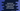# Typescript concat and split explanation with example# Introduction :

In this tutorial, we will learn how to split a string and how to concatenate multiple sub-strings in typescript. Typescript provides two different methods to do the concatenate and splitting easily. Let’s have a look :

## Concat :

concat method in typescript is used to concatenate multiple sub-strings.The concate method is defined as below :

``````str.concat(str1[,str2,str3,....])
``````

### Arguments :

concat method takes one or more sub-strings as arguments. These are the strings that it will concatenate.

### Return value :

This method returns one new string i.e. the final string. For example :

### Example program :

``````let helloStr = "Hello";
let worldStr = "World";
let blankStr = " ";

let finalStr = helloStr.concat(blankStr,worldStr);
console.log(`Final string : \${finalStr}`);
``````

### Output :

``````Final string : Hello World
``````

## Split :

split method is used to split one string into an array of substrings. This method is defined as below :

``````str.split(separator[,limit])
``````

### Arguments :

It takes two parameters. separator is the split separator character. And limit is the total number of substrings required in the final array.

### Return value :

This method returns an array of substrings. For example :

### Example program :

``````let str : string = "one,two,three,four,five,six";

let arr1 : string[] = str.split(",");
let arr2 : string[] = str.split(",",4);

console.log(`arr1 : \${arr1}`);
console.log(`arr2 : \${arr2}`);
``````

It will print :

``````arr1 : one,two,three,four,five,six
arr2 : one,two,three,four
``````

Both are arrays here. The second console.log prints the first four elements.